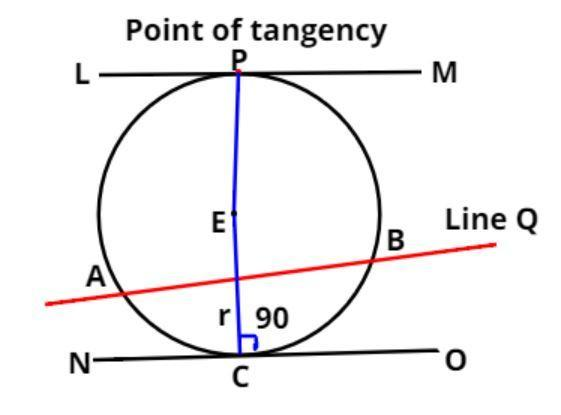Courses
Courses for Kids
Free study material
Free LIVE classes
MoreLIVE
Join Vedantu’s FREE Mastercalss

# Fill in the blanks:$\left( i \right)$ The common point of a tangent and the circle is called…………..$\left( {ii} \right)$ A circle may have …………….. Parallel tangents.$\left( {iii} \right)$ A tangent to a circle intersect in it …………….. Point(s).$\left( {iv} \right)$ A line intersecting a circle in two points is called a……………..$\left( v \right)$ The angle between tangent at a point on a circle and the radius through the point is……….Verified
362.4k+ views
Hint: In this question first draw the diagram it will give us a clear picture of what we have to find out later on using the property that the radius of the circle is always perpendicular to the tangent at the point of intersection so, use this concept to reach the solution of the question.Let us consider the circle as above with center E and radius r as shown in figure.
EC = r
Let the diameter of the circle be PC.
The tangents of the circle are LM and NO (see figure).
Let the points at which the tangents LM and NO intersect are P and C respectively (see figure).
Let us consider a line Q which intersects the circle at points A and B respectively.
$\left( i \right)$ The common point of a tangent and the circle is called…………..
The common point of a tangent and the circle is called point of tangency (see figure at point P).
$\left( {ii} \right)$ A circle may have …………….. Parallel tangents.
A circle can have exactly two parallel tangents and they must pass through the ends of the diameter of the circle PC (see figure) and the parallel tangents are LM and NO.
$\left( {iii} \right)$ A tangent to a circle intersect in it …………….. Point(s).
A tangent to a circle intersects at only a single point.
For example for tangent LM it intersects the circle at point P (see figure), and for tangent NO it intersects the circle at point C (see figure).
$\left( {iv} \right)$ A line intersecting a circle in two points is called a……………..
When a line intersects the circle at two points is called a chord of the circle.
For example line Q intersects the circle at point A and B (see figure) which is the chord of the circle.
$\left( v \right)$ The angle between tangents at a point on a circle and the radius through the point is……….
As we all know the radius of the circle is always passing through the center of the circle therefore radius r and tangent NO intersect at point P (see figure).
As we all know radius is always perpendicular to the tangent so it makes a 90 degree with the tangent at the point of intersection.
$\Rightarrow \angle ECO = {90^0}$ (See figure).

Note: In such types of questions the key concept we have to remember is that point of contact of the tangent with the circle is point of tangency, a circle has 2 parallel tangents and it must pass through the end points of the diameter, a tangent to the circle is always intersect at a single point, chord of the circle is always intersect the circle at two points, and radius is always perpendicular to the tangent so it makes a 90 degree with the tangent at the point of intersection.
Last updated date: 24th Sep 2023
Total views: 362.4k
Views today: 9.62k